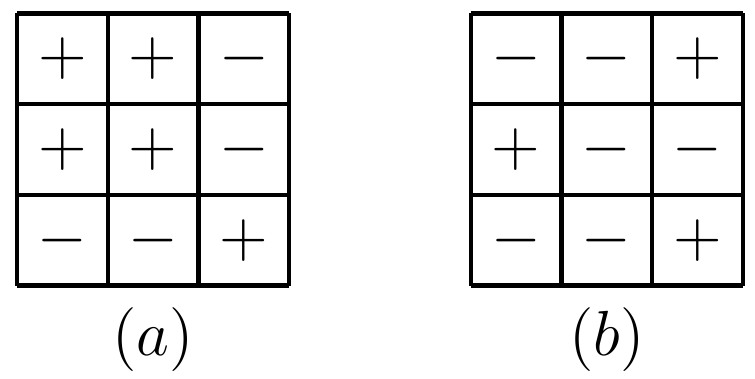#### Invariant

Obtaining the correct answer is not the sole purpose of practice. Another equally, if not more important objective is to check whether we can truly understand the nature of a problem and quickly identify the most appropriate solving technique. This is more useful and beneficial than merely being able to solve a particular practice problem.

Let's review a daily question presented on 2017/10/02.

 (3834) There are three piles which contain $8$, $9$, and $19$ stones, respectively. You are allowed to choose two piles and transfer one stone from each of them to the third pile. Is it possible to make all piles all contain exactly $12$ stones after several such operations?

This is a typical invariant problem which is often associated with parity analysis/modular arithmetic (MOD) in number theory.

Invariant means something remains unchanged during a series of transformation. In this problem, transformation is clearly the movement of stones. What we need to find out is which property will remain the same regardless of such transformation.

For basic to intermediate level problems, an invariant property usually is related to odd-even parity or MOD. In this particular case, it is the set of remainders when the numbers of stones in each pile are divided by $3$. Or

$$Invariant,MOD(3)$$

It can be easily verified that the remainders will always be $(0,1,2)$ regardless of how many times stones are moved among piles. This means the target status cannot be reached because it requires the remainders to be $(0,0,0)$.

Invariant is discussed in more detail in the book . Here is a slightly trickier example from this book.

 (3927) $\textbf{Flip the Grid}$Given two grids shown below, is it possible to transform ($a$) to ($b$) after a series of operations? In each operation, one can change all the signs in either one entire row or one entire column.